# Differential Equations in AP Calculus: Help and Review Chapter Exam

Exam Instructions:

Choose your answers to the questions and click 'Next' to see the next set of questions. You can skip questions if you would like and come back to them later with the yellow "Go To First Skipped Question" button. When you have completed the practice exam, a green submit button will appear. Click it to see your results. Good luck!

### Page 1

#### Question 1 1. Solve the following differential equation for y=f(x) (assume that y is always positive).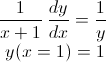#### Question 2 2. Solve the following differential equation for c=f(a).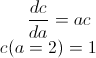#### Question 3 3. You have a tank that is shaped like a prism that is on its side. The triangular base of the tank is a right triangle of height 10 m and width 10 m. See the image below. If the tank is being filled at a rate of 30 cubic meters per minute, how quickly is the height changing?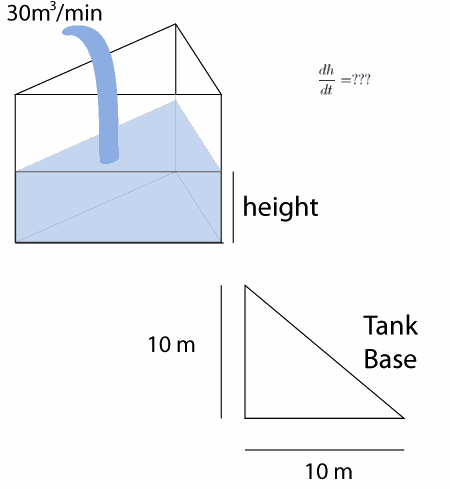#### Question 5 5. Solve the following differential equation for y=f(x).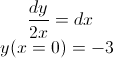### Page 2

#### Question 6 6. What is the equation for L as a function of x?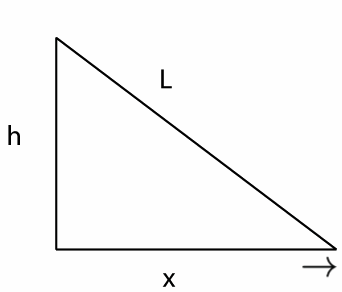### Page 3

#### Question 12 12. If h equals 3 m, and x is moving at a rate of 3 m / second, how fast is L changing when x equals 4m?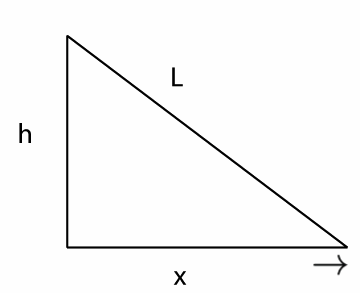#### Question 14 14. Solve the differential equation for your bank balance given as follows.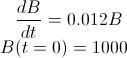### Page 4

#### Question 19 19. What is the equation of how L changes as a function of how x changes if h=6?#### Question 20 20. The red and blue cars are 10 miles apart, but headed toward one another. The red car is traveling at 45 mph, and the blue car is traveling at a speed v(t). What is the equation for how the distance between them changes as a function of time?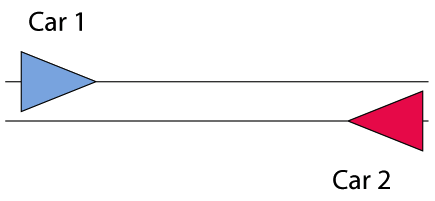### Page 5

#### Question 21 21. Solve the following differential equation for y=f(x).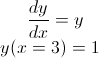#### Question 22 22. If the red car is traveling at 45 mph, and the blue is braking so that v(t) = 60 - 30t, then when will they pass each other, assuming that they are 10 miles apart at t = 0?#### Question 23 23. Solve the following differential equation for y=f(x) (assume that y is always positive).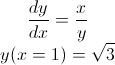#### Question 24 24. The following differential equation represents the change in population as a function of time. What does the 0.03 represent in this equation?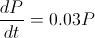#### Differential Equations in AP Calculus: Help and Review Chapter Exam Instructions

Choose your answers to the questions and click 'Next' to see the next set of questions. You can skip questions if you would like and come back to them later with the yellow "Go To First Skipped Question" button. When you have completed the practice exam, a green submit button will appear. Click it to see your results. Good luck!

Support